Doubly Stochastic Matrices

Constrained Optimization

Generate random matrix, find closest doubly stochastic in least square sense

In:=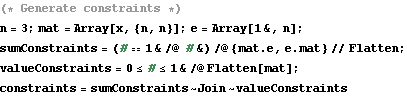Out=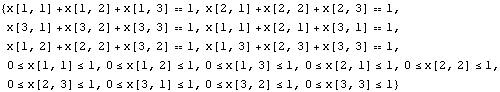In:=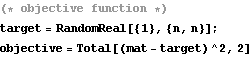Out=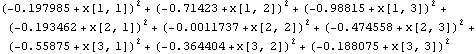In:=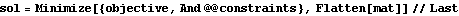Out=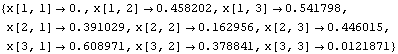In:=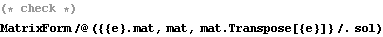Out=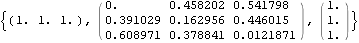Markov Chain sampling

Start with a random permutation matrix, randomly modify 4 entries each time

In:=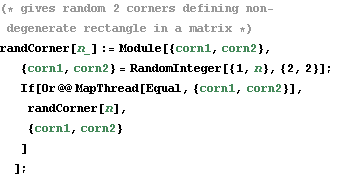In:=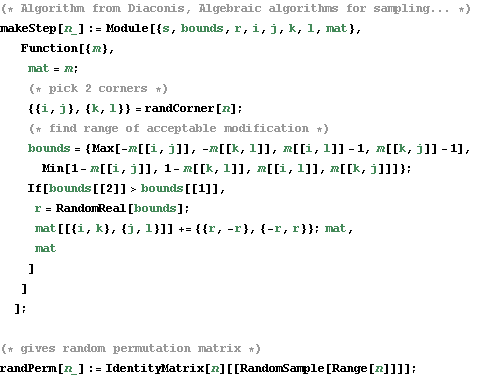In:=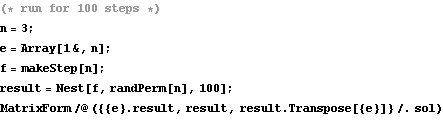Out=Created by Wolfram Mathematica 6.0  (17 December 2007)